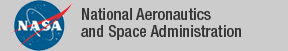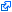### Formalization of Tarski's Theorem

Tarski's Theorem is a generalization of Sturm's theorem that provides a linear relationship between functions known as Tarski queries and cardinalities of certain sets. The linear system that results from this relationship is in fact invertible and can be used to explicitly count the number of roots of a univariate polynomial on a set defined by a system of polynomial relations. This computation is used to define a computable function that calculates whether any system of univariate polynomials is satisfiable. The PVScontribution `Tarski`, which is part of the NASA PVS Library, includes a formalization of Tarski's Theorem, as well as a decision procedure that determines the satisfiability of a system of univariate polynomial relations over the real line. It is formally verified in PVS that the decision procedure is sound and complete. This result is a the basis of the proof-producing strategy `tarski` for reasoning about systems of univariate polynomial relations on the real line. The soundness of the strategy depends solely on the internal logic of PVS rather than on an external oracle.

### PVS Contribution

• Formalization of Tarski's theorem and PVS strategies (see dependency graph).
• Strategy `tarski`: Automatically discharges systems of polynomial relations.
• Examples.
• Sturm's Theorem.
• ### Publications

The tagidentifies links that are outside the NASA domain